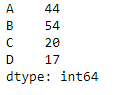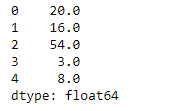# Python | Pandas dataframe.max()

• Last Updated : 19 Nov, 2018

Python is a great language for doing data analysis, primarily because of the fantastic ecosystem of data-centric python packages. Pandas is one of those packages and makes importing and analyzing data much easier.

Pandas` dataframe.max()` function returns the maximum of the values in the given object. If the input is a series, the method will return a scalar which will be the maximum of the values in the series. If the input is a dataframe, then the method will return a series with maximum of values over the specified axis in the dataframe. By default the axis is the index axis.

Attention geek! Strengthen your foundations with the Python Programming Foundation Course and learn the basics.

To begin with, your interview preparations Enhance your Data Structures concepts with the Python DS Course. And to begin with your Machine Learning Journey, join the Machine Learning - Basic Level Course

Syntax: DataFrame.max(axis=None, skipna=None, level=None, numeric_only=None, **kwargs)

Parameters :
axis : {index (0), columns (1)}
skipna : Exclude NA/null values when computing the result
level : If the axis is a MultiIndex (hierarchical), count along a particular level, collapsing into a Series
numeric_only : Include only float, int, boolean columns. If None, will attempt to use everything, then use only numeric data. Not implemented for Series.

Returns : max : Series or DataFrame (if level specified)

Example #1: Use `max()` function to find the maximum value over the index axis.

 `# importing pandas as pd``import` `pandas as pd`` ` `# Creating the dataframe ``df ``=` `pd.DataFrame({``"A"``:[``12``, ``4``, ``5``, ``44``, ``1``],``                   ``"B"``:[``5``, ``2``, ``54``, ``3``, ``2``],``                   ``"C"``:[``20``, ``16``, ``7``, ``3``, ``8``], ``                   ``"D"``:[``14``, ``3``, ``17``, ``2``, ``6``]})`` ` `# Print the dataframe``df`Let’s use the `dataframe.max()` function to find the maximum value over the index axis

 `# Even if we do not specify axis = 0, ``# the method will return the max over``# the index axis by default``df.``max``(axis ``=` `0``)`

Output :Example #2: Use `max()` function on a dataframe which has `Na` values. Also find the maximum over the column axis.

 `# importing pandas as pd``import` `pandas as pd`` ` `# Creating the dataframe ``df ``=` `pd.DataFrame({``"A"``:[``12``, ``4``, ``5``, ``None``, ``1``], ``                   ``"B"``:[``7``, ``2``, ``54``, ``3``, ``None``],``                   ``"C"``:[``20``, ``16``, ``11``, ``3``, ``8``],``                   ``"D"``:[``14``, ``3``, ``None``, ``2``, ``6``]})`` ` `# skip the Na values while finding the maximum``df.``max``(axis ``=` `1``, skipna ``=` `True``)`

Output :My Personal Notes arrow_drop_up